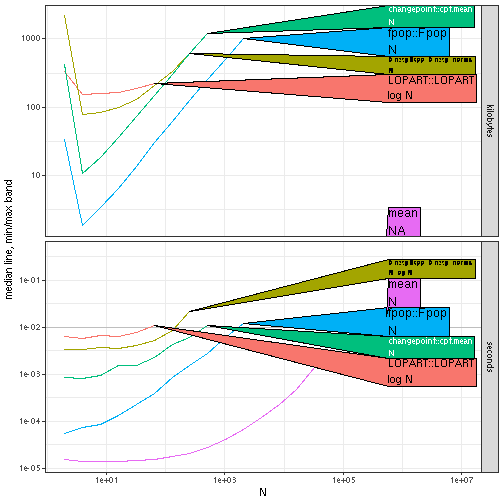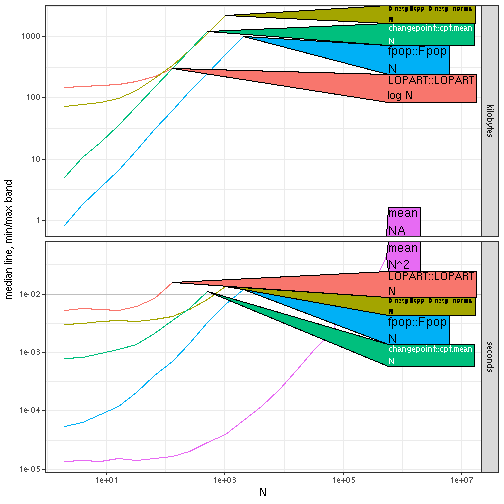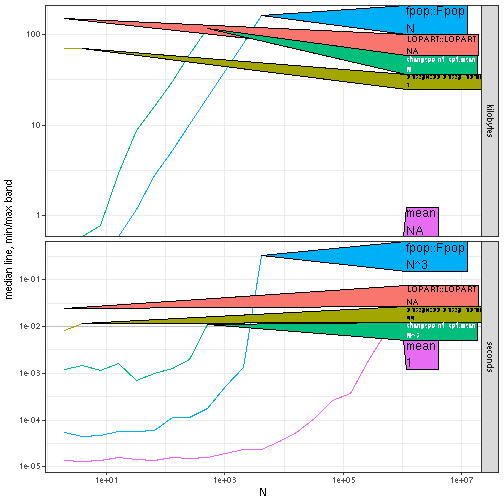# Custom Plots

The goal of this vignette is to demonstrate how to create custom ggplots (instead of always using the `plot` method). In particular, we would like to display the best fit asymptotic complexity class in the direct label, along side the expression name. We use optimal segmentation algorithms as an example.

``````library(data.table)
viz.data <- function(get.seg.means, data.per.seg=10, penalty=1){
no.labels <- data.table(
start=integer(), end=integer(), changes=integer())
expr.list <- c(
if(requireNamespace("changepoint"))atime::atime_grid(
"changepoint::cpt.mean"=changepoint::cpt.mean(
data.vec, method="PELT", penalty="Manual", pen.value=penalty)),
if(requireNamespace("binsegRcpp"))atime::atime_grid(
"binsegRcpp::binseg_normal"=binsegRcpp::binseg_normal(data.vec, N)),
if(requireNamespace("fpop"))atime::atime_grid(
"fpop::Fpop"=fpop::Fpop(data.vec, penalty)),
if(requireNamespace("LOPART"))atime::atime_grid(
"LOPART::LOPART"=LOPART::LOPART(data.vec, no.labels, penalty)),
atime::atime_grid(mean=mean(data.vec)))
atime.list <- atime::atime(
N=2^seq(1, 20),
setup={
seg.means <- get.seg.means(N)
mean.vec <- rep(seg.means, each=data.per.seg)
set.seed(1)
data.vec <- rnorm(data.per.seg*N, mean.vec, 0.2)
},
expr.list=expr.list,
times=5)
best.list <- atime::references_best(atime.list)
if(require(ggplot2)){
hline.df <- with(atime.list, data.frame(seconds.limit, unit="seconds"))
gg <- ggplot()+
theme_bw()+
facet_grid(unit ~ ., scales="free")+
geom_hline(aes(
yintercept=seconds.limit),
color="grey",
data=hline.df)+
geom_line(aes(
N, empirical, color=expr.name),
data=best.list\$meas)+
geom_ribbon(aes(
N, ymin=min, ymax=max, fill=expr.name),
data=best.list\$meas[unit=="seconds"],
alpha=0.5)+
scale_x_log10()+
scale_y_log10("median line, min/max band")
if(require(directlabels)){
gg+
directlabels::geom_dl(aes(
N, empirical, color=expr.name, label=expr.class),
method="right.polygons",
data=best.list\$meas)+
theme(legend.position="none")+
coord_cartesian(xlim=c(2,1e7))
}else{
gg
}
}
}
viz.data(function(N.segs)rep(0:1,l=N.segs))
#> Warning: Transformation introduced infinite values in continuous y-axis
#> Transformation introduced infinite values in continuous y-axis
#> Warning in grid.Call.graphics(C_polygon, x\$x, x\$y, index): semi-transparency is
#> not supported on this device: reported only once per page
``````The plots above show some speed differences between segmentation algorithms. It is clear that LOPART and binseg algorithms are slow/quadratic in these data (ten points up, ten points down, ten points up, …), whereas FPOP and PELT (changepoint pkg) are fast/log-linear. This is the worst case for binseg.

``````viz.data(function(N.segs)1:N.segs)
#> Warning: Transformation introduced infinite values in continuous y-axis
#> Transformation introduced infinite values in continuous y-axis
#> Warning in grid.Call.graphics(C_polygon, x\$x, x\$y, index): semi-transparency is
#> not supported on this device: reported only once per page
``````Results above show a more typical result: LOPART is slow/quadratic whereas others are fast/log-linear.

``````viz.data(function(N.segs)1:N.segs, data.per.seg=1, penalty=1e10)
#> Warning: Transformation introduced infinite values in continuous y-axis
#> Transformation introduced infinite values in continuous y-axis
#> Warning in grid.Call.graphics(C_polygon, x\$x, x\$y, index): semi-transparency is
#> not supported on this device: reported only once per page
``````Results above show a highly unusual/pathological result: FPOP and PELT are quadratic time for a data sequence which is always increasing with a large penalty.